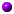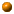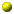Lecture notes for Math 55a: Honors Advanced Calculus and Linear Algebra (Fall 2005)

If you find a mistake, omission, etc., please let me know by e-mail.

The orange balls mark our current location in the course, and the current problem set.Ceci n'est pas un Math 55a syllabus (PS [PostScript] or PDF)Our first topic is the topology of metric spaces, a fundamental tool of modern mathematics that we shall use mainly as a key ingredient in our rigorous development of differential and integral calculus. To supplement the treatment in Rudin's textbook, I wrote up 20-odd pages of notes in six sections; copies will be distributed in class, and you also may view them and print out copies in advance from the PostScript or PDF files linked below.Metric Topology I (PS, PDF)
Basic definitions and examples: the metric spaces Rn and other product spaces; isometries; boundedness and function spacesIf S is an infinite set and X is an unbounded metric space then we can't use our definition of XS as a metric space because supS dX(f(s),g(s)) might be infinite. But the bounded functions from S to X do constitute a metric space under the same definition of dXS. A function is said to be bounded'' if its image is a bounded set. You should check that that dXS(f,g) is in fact finite for bounded f and g.The Proposition'' on page 3 of the first topology handout can be extended as follows:
iv) For every point p of X there exists a real number M such that d(p,q)<M for all q of E.
In other words, for every p in X there exists an open ball about p that contains E. Do you see why this is equivalent to (i), (ii), and (iii)?Metric Topology II (PS, PDF)
Open and closed sets, and related notions
corrected 22.ix.05 (p.2: indices changed from i to alpha in Thm. 2.24 parts a,b;
p.4: closure of Br(p) vs. closed ball of radius r, also fixed typo subet'' for subset)Metric Topology III (PS, PDF)
Introduction to functions and continuity
corrected 25.ix.05 (V for Z five times in p.3, paragraph 2)Metric Topology IV (PS, PDF)
Sequences and convergence, etc.Metric Topology V (PS, PDF)
Compactness and sequential compactness
corrected 1.x.05 to fix various minor typosMetric Topology VI (PS, PDF)
Cauchy sequences and related notions (completeness, completions, and a third formulation of compactness)at least in the beginning of the linear algebra unit, we'll be following the Axler textbook closely enough that supplementary lecture notes should not be needed. Some important extensions/modifications to the treatment in Axler:
• [cf. Axler, p.3] Unless noted otherwise, F may be an arbitrary field, not only R or C. The most important fields other than those of real and complex numbers are the field Q of rational numbers, and the finite fields Z/pZ (p prime). Other examples are the field Q(i) of complex numbers with rational real and imaginary parts; more generally, Q(d1/2) for any nonsquare rational number d; the p-adic numbers'' Qp (p prime), introduced at the end of our topology unit; and more exotic finite fields such as the 9-element field (Z/3Z)(i). Here's a review of the axioms for fields, vector spaces, and related mathematical structures.
• [cf. Axler, p.22] We define the span of an arbitrary subset S of (or tuple in) a vector space V as follows: it is the set of all (finite) linear combinations a1 v1 + ... + an vn with each vi in V and each ai in F. This is still the smallest vector subspace of V containing S. In particular, if S is empty, its span is by definition {0}. We do not require that S be finite.
• About the infamous Lemma 3.?'': some notes and warnings about the behavior of Hom(V,W) under (finite or infinite) direct sums.
• Unlike Axler, we spend some time on quotient vector spaces''.
• Axler also unaccountably soft-pedals the important notion of duality.
• Here's a brief preview of abstract nonsense (a.k.a. diagram-chasing), and a diagram-chasing interpretation of quotients and duality.
• Axler proves the Fundamental Theorem of Algebra using complex analysis, which cannot be assumed in Math 55. Here's a proof using the topological tools we developed in the first month of class, in PS and PDF. (Axler gives the complex-analytic proof on page 67.)
• We shall need some eigenstuff'' also in an infinite-dimensional setting, so will not assume that any vector space is (nonzero) finite dimensional unless we really must.
• If T is a linear operator on a vector space V, and U is an invariant subspace, then the quotient space V/U inherits an action of T. Moreover, the annihilator of U in V* is an invariant subspace for the action of the adjoint operator T* on V*. (Make sure you understand why both these claims hold.)
• Triangular matrices are intimately related with flags''. A flag in a finite dimensional vector space V is a sequence of subspaces {0}=V0, V1, V2, ..., Vn=V, with each Vi of dimension i and containing Vi-1. A basis v1, v2, ..., vn determines a flag: Vi is the span of the first i basis vectors. Another basis w1, w2, ..., wn determines the same flag if and only if each wi is a linear combination of v1, v2, ..., vi (necessarily with nonzero vi coefficient). The standard flag in Fn is the flag obtained in this way from the standard basis of unit vectors e1, e2, ..., en. The punchline is that, just as a diagonal matrix is one that respects the standard basis (equivalently, the associated decomposition of V as a direct sum of 1-dimensional subspaces), an upper-triangular matrix is one that respects the standard flag. Note that the i-th diagonal entry of a triangular matrix gives the action on the one-dimensional quotient space Vi/Vi-1 (each i=1,...,n).Less surprising than the absence of quotients and duality in Axler is the lack of tensor algebra. That won't stop us in Math 55, though. Here's an introduction in PS and PDF. [As you might guess from \oplus, the TeXism for the tensor-product symbol is \otimes.]
• One of many applications is the trace of an operator on a finite dimensional F-vector space V. This is a linear map from Hom(V,V) to F. We can define it simply as the composition of two maps: our identification of Hom(V,V) with the tensor product of V* and V, and the natural map from this tensor product to F coming from the bilinear map taking (v*,v) to v*(v).
• Here are some basic facts about general norms on real and complex vector spaces.
• Just as we can study bilinear symmetric forms on a vector space over any field, not just R, we can study sesquilinear conjugate-symmetric forms on a vector space over any field with a conjugation, not just C. Here a conjugation'' on a field F is a field automorphism sigma:F-->F such that sigma is not the identity but sigma2 is (that is, sigma is an involution). Given a basis {vi} for F, a sesquilinear form <.,.> on F is determined by the field elements ai,j=<vi,vj>, and is conjugate-symmetric if and only if aj,i=sigma(ai,j) for all i,j. Note that the diagonal entries'' ai,i --- and more generally <v,v> for any v in V --- must be elements of the subfield of F fixed by sigma.
• Sylvester's Law of Inertia'' states that for a nondegenerate pairing on a finite-dimensional vector space V/F, where either F=R and the pairing is bilinear and symmetric, or F=C and the pairing is sesquilinear and conjugate-symmetric, the counts of positive and negative inner products for an orthogonal basis constitute an invariant of the pairing and do not depend on the choice of orthogonal basis. (This invariant is known as the signature'' of the pairing.) The key trick in proving this result is as follows. Suppose V is the orthogonal direct sum of subspaces U1, U2 for which the pairing is positive definite on U1 and negative definite on U2. Then any subspace W of V on which the pairing is positive definite has dimension no greater than dim(U1). Proof: On the intersection of W with U2, the pairing is both positive and negative definite; hence that subspace is {0}. The claim follows by a dimension count, and we quickly deduce Sylvester's Law.
• Over any field not of characteristic 2, we know that for any non-degenerate symmetric pairing on a finite-dimensional vector space there is an orthogonal basis, or equivalently a choice of basis such that the pairing is (x,y)=sumi(ai xi yi) for some nonzero scalars ai. But in general it can be quite hard to decide whether two different collections of ai yield isomorphic pairings. Even over Q the answer is already tricky in dimensions 2 and 3, and I don't think it's known in a vector space of arbitrary dimension.
• Re Chapter 7: we'll prove the Spectral Theorem for a self-adjoint or Hermitian operator T on a finite-dimensional real or complex vector space, not a la Axler, but by the usual method of maximizing the Rayleigh quotient'' <Tv,v>/<v,v>. Likewise for normal operators on a finite dimensional C-vector space, by maximizing <Tv,Tv>/<v,v>.
• All of Chapter 8 works over an arbitrary algebraically closed field, not only over C (except for the minor point about extracting square roots, which breaks down in characteristic 2); and the first section (Generalized Eigenvalues'') works over any field.
• We don't stop at Corollary 8.8: let T be any operator on a vector space V over a field F, not assumed algebraically closed. If V is finite-dimensional, then The Following Are Equivalent:
(1) There exists a nonnegative integer k such that Tk=0;
(2) For any vector v, there exists a nonnegative integer k such that Tkv=0;
(3) Tn=0, where n=dim(V).
Note that (1) and (2) make no mention of the dimension, but are still not equivalent for operators on infinite-dimensional spaces. We readily deduce the further equivalent conditions:
(4) There exists a basis for V for which T has an upper-triangular matrix with every diagonal entry equal zero;
(5) Every upper-triangular matrix for T has zeros on the diagonal, and there exists at least one upper-triangular matrix for T.
Recall that the second part of (5) is automatic if F is algebraically closed.
• The space of generalized 0-eigenvectors (the maximal subspace on which T is nilpotent) is sometimes called the nilspace of T. It is an invariant subspace. When V is finite dimensional, V is the direct sum of the nilspace and another invariant subspace V', consisting of the intersection of the subspaces Tk(V) as k ranges over all positive integers. See Exercise 8.11; This can be used to quickly prove Theorem 8.23 and consequences such as Cayley-Hamilton (Theorem 8.20).
• The dimension of the space of generalized c-eigenvalues (i.e., of the nilspace of T-cI) is usually called the algebraic multiplicity of c (since it's the multiplicity of c as a root of the characteristic polynomial of T), to distinguish it from the geometric multiplicity'' which is the dimension of ker(T-cI).We'll define the determinant of an operator T on a finite dimensional space V as follows: T induces a linear operator T' on the top exterior power of V; this exterior power is one-dimensional, so an operator on it is multiplication by some scalar; det(T) is by definition the scalar corresponding to T'. The top exterior power'' is a subspace of the exterior algebra'' of V, which is the quotient of the tensor algebra by the ideal generated by {v*v: v in V}. We'll still have to construct the sign homomorphism from the symmetry group of order dim(V) to {1,-1} to make sure that this exterior algebra is as large as we expect it to be, and that in particular that the (dim(V))-th exterior power has dimension 1 rather than zero.

Some more tidbits about exterior algebra:

• If w, w' are elements of the m-th and m'-th exterior powers of V, then ww'=(-1)mm'w'w; that is, w and w' commute unless m and m' are both odd in which case they anticommute.
• If m+m'=n=dim(V) then the natural pairing from the m-th and m'-th exterior powers to the n-th is nondegenerate, and so identifies the m'-th exterior power canonically with the dual of the m-th tensored with the top (n-th) exterior power.
• In particular, if m=1, and T is any invertible operator on V, then we find that the induced action of T on the (n-1)st exterior power is the same as its action on V* multiplied by det(T). This yields the formula connecting the inverse and cofactor matrix of an invertible matrix (a formula which you may also know in the guise of Cramer's rule'').
• For each m there is a natural non-degenerate pairing between the m-th exterior powers of V and V*, which identifies these exterior powers with each other's dual.
We'll also show that a symmetric (or Hermitian) matrix is positive definite iff all its eigenvalues are positive iff it has positive principal minors (the principal minors'' are the determinants of the square submatrices of all orders containing the (1,1) entry). More generally we'll show that the eigenvalue signs determine the signature, as does the sequence of signs of principal minors. For positive definiteness, we have the two further equivalent conditions: the symmetric (or Hermitian) matrix A=(aij) is positive definite iff there is a basis (vi) of Fn such that aij=<vi,vj> for all i,j, and iff there is an invertible matrix B such that A=BB*. For example, the matrix with entries 1/(i+j-1) (Hilbert matrix'') is positive-definite, because it is the matrix of inner products (integrals on [0,1]) of the basis 1,x,x2,...,xn-1 for the polynomials of degree <n. Can you find the determinant of this matrix?

More will be said about exterior algebra when differential forms appear in Math 55b.First & second problem set: Metric topology (PS, PDF)
corrected 19.ix.05 (clarification in 4(i), typos in 4(iv) and 11, parenthetical addendum in 11)
corrected again 20.ix.05: #6(ii*) had the wrong condition! :-( Shrenik Shah was the first to show it was impossible as first stated.Third problem set: Metrics, sequences, compactness, and a bit of general topology (PS, PDF)Fourth problem set: Topology grand finale (PS, PDF)Fifth problem set / Linear Algebra I: vector space basics (PS, PDF)Sixth problem set / Linear Algebra II: the dimension and some of its uses (PS, PDF)Seventh problem set / Linear Algebra III: linear maps and duality (PS, PDF)Eighth problem set / Linear Algebra IV: Eigenstuff, and a projective overture (PS, PDF)
corrected 9.xi.05 (typo in 1/iii)Ninth problem set / Linear Algebra V: Tensors, etc. (PS, PDF)Tenth problem set / Linear Algebra VI: Inner products, lattices, and normal operators (PS, PDF)Here's my solution for problem 4 (and 3) (PS, PDF)Eleventh problem set / Linear Algebra VII: Fourier foretaste, and symplectic structures (PS, PDF)Twelfth and last problem set / Linear Algebra VIII: Groups, exterior algebra, and determinants (PS, PDF)
corrected 15.xii.05 (vi, not ||vi||, in 6(i); typo in 7 -- thanks to Scott K. for both;
also in 7, a sentence to say explicitly that k is fixed throughout;
and in the introductory paragraph for 8, the pairing is on V*, not V, and the map is from V* to V, not V to V*);
and (16.xii.05) again in 8, the associated pairing'' is now specified.